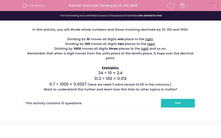# Decimals: Dividing by 10, 100, 1000

In this worksheet, students divide whole numbers and those involving decimals by 10, 100 and 1000.This content is premium and exclusive to EdPlace subscribers.Key stage:  KS 2

Curriculum topic:   Maths and Numerical Reasoning

Curriculum subtopic:   Decimals

Difficulty level:#### Worksheet Overview

In this activity, you will divide whole numbers and those involving decimals by 10, 100 and 1000.

Dividing by 10 moves all digits one place to the right.

Dividing by 100 moves all digits two places to the right.

Dividing by 1000 moves all digits three places to the right and so on.

Remember that when a digit moves from the units place to the tenths place, it hops over the decimal point.

Examples:

24 ÷ 10 = 2.4
31.2 ÷ 100 = 0.312
0.7 ÷ 1000 = 0.0007
(Here we need 3 extra zeroes to fill in the columns.)

Want to understand this further and learn how this links to other topics in maths?

### What is EdPlace?

We're your National Curriculum aligned online education content provider helping each child succeed in English, maths and science from year 1 to GCSE. With an EdPlace account you’ll be able to track and measure progress, helping each child achieve their best. We build confidence and attainment by personalising each child’s learning at a level that suits them.

Get started